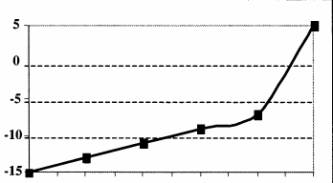•November 30, 2023<< November 2023 >>S M T W T F S 1 2 3 4 5 6 7 8 9 10 11 12 13 14 15 16 17 18 19 20 21 22 23 24 25 26 27 28 29 30Important LinksFollow Us!•  SAMPLE ALGEBRA AND FUNCTIONS
 Nov 12, 2019

This is a test of your ability to solve problems using algebra. There are three (3) sample questions.

1. Consider the following formula:
A = B + 3 ( 4 - C )

If B equals 5 and C equals 2, what is the value of A?
A.7
B.11
C.12
D.17

2. Consider the following formula:
y = 3 ( x + 5 ) ( x - 2 )

Which of the following formulas is equivalent to this one?
A. y=3x2+9x-30
B. y=x2+3x-lO
C. y=3x2+3x-lO
D. y=3x2+3x-30

3. Consider the following pattern of numbers:
110, 112, 107, 109, 104

What is the next number in the pattern?
A.97
B. 99
C. 106
D.109

4. Consider the following formula:
a = 1/2 b - 4

Which of the following statements is true for this formula?
A. When the value of is less than 8, a is negative.
B. When the value of is greater than 8, a is negative.
C. When the value of is less than 8, a is positive.
D. When the value of is greater than 4, a is positive.

5. Consider the following table:  X Y 0 -5 1 -4 2 -3 3 -2 4 -1 5 0 6 1

Which of the following choices represents the same relationship as demonstrated in this table?

 A. X Y 10 -40 20 -30 30 -20 40 -10

B. = x/2 - 5

C. is equivalent to the difference between the value of X and a constant C, where equals 5.

 D. Y-20 -19 -18 -17 -16 -15 -14 -13 -12 -11 -10 X